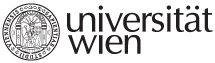Name of Course DXE_EMT2 Econometrics 2 Lecturer Prof. Dr. Peter Hackl Assistant Ing. Daniel Němec, Ph.D. ECTS 12 credits Extent and Intensity 24 teaching hours, 45 minutes each (6 lectures á 3 hours) Schedule 9. 3 ., 16. 3., 23. 3., 6. 4., 13. 4., 20. 4.; 10:00 - 14:00 Course objectives On the basis of the introduction to econometrics as covered in the course Econometrics, advanced econometric topics will be the subject of this course. Besides conceptual topics such as maximum likelihood estimation, econometric methods for empirical analysis of time series data will be the main content; in addition, models for analyzing limited dependent variables and for analyzing panel data will be covered. The course is designed to provide students with a good knowledge of basic and advanced econometric tools so that: - they will be able to apply these tools to modeling, estimation, inference, and forecasting in the context of economic problems; - they have experience in applying the econometric software Gretl for analyzing data; - they can evaluate critically the results from others who use econometric methods and tools; - they have a basis for further studies of econometric literature. Prerequisites Participants should be familiar with the following topics: Linear algebra – linear equations, matrices, vectors (basic operations and properties). Descriptive statistics – measures of central tendency, measures of dispersion, measures of association, histogram, frequency tables, scatterplot, quantiles. Theory of probability – probability and its properties, random variables and distribution functions in one and several dimensions, moments, convergence of random variables, limit theorems, law of large numbers. Mathematical statistics – point estimation, confidence intervals for parameters of normal distribution, hypothesis testing, p-value, significance level. Basic econometrics - ordinary least squares method, linear regression, classical assumptions and their violations. These topics correspond to the chapters the appendices of Verbeek’s book, in particular, to the chapters 1-5 and sections: A1, A2, A3, A4, A6, A8, B1, B2, B3 (excluding Jensen's inequality), B4, B5, B6 and B7 (excluding some properties of the chi-squared distribution and the F-distribution). Syllabus 1. Maximum likelihood Estimation and Specification Tests (Verbeek, Ch.6)     - The concept of maximum likelihood;     - Specification tests;     - Tests in the normal linear regression model;     - Quasi-maximum likelihood and moment conditions tests. 2. Models with Limited Dependent Variables (Verbeek, Ch.7)     - Binary choice models;     - Multiresponse models;     - Tobit models.  3. Univariate time series models (Verbeek, Ch.8)     - ARMA processes;     - Stationarity and unit roots;     - Testing for unit roots;     - Estimation of ARMA models;     - Choosing a model. 4. Multivariate time series models, part 1 (Verbeek, Ch.9)     - Predicting with ARMA models;     - Autoregressive conditional heteroskedasticity;     - Dynamic models with stationary variables;     - Models with nonstationary variables. 5. Multivariate time series models, part 2 (Verbeek, Ch.9)     - Vector autoregressive models;     - Cointegration: the multivariate case; 6. Models Based on Panel Data (Verbeek, Ch.10)     - Static and dynamic linear models;     - Nonstationarity, unit roots, and cointegration;     - Models with limited dependent variables. Literature VERBEEK, Marno. A guide to modern econometrics. 3rd ed. Chichester: John Wiley & Sons, 2008. xv, 472. ISBN 9780470517697. Teaching methods Class discussion, homework including computer exercises using Gretl, and presentation of homework by participants; course language is English. Assessment methods For grading, written homework, presentation of homework in class, and a final written exam will be taken into account. The weight for homework will be 40 %, that of the written final exam 60 %. Presentation of homework in class means that students must be prepared to be called at random to the blackboard. Additional notes The minimum of students for opening the course is 5.
University of Vienna | Universitätsring 1 | 1010 Vienna | T +43-1-4277-0